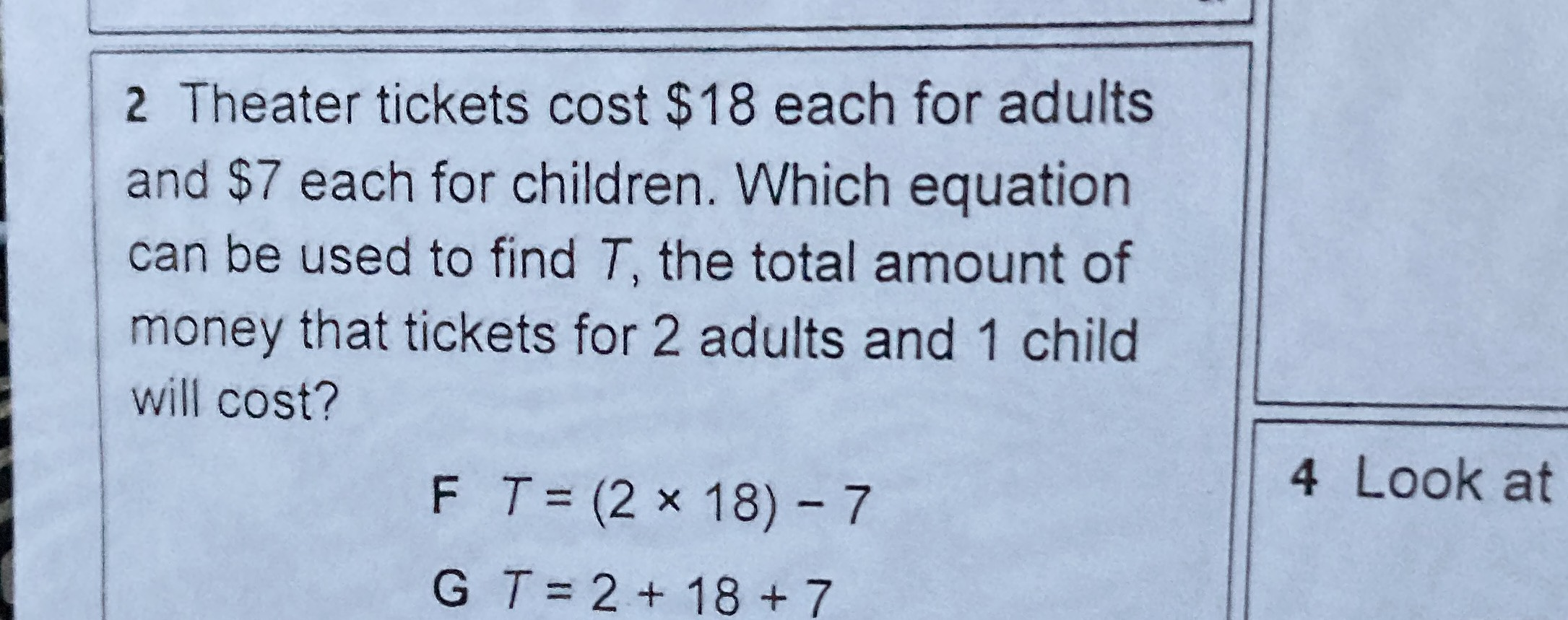### ¿Todavía tienes preguntas de matemáticas?

Pregunte a nuestros tutores expertos
Algebra
Pregunta$$2$$ Theater tickets cost $$\ 18$$ each for adults and $$\ 7$$ each for children. Which equation can be used to find $$T$$ , the total amount of money that tickets for $$2$$ adults and $$1$$ child will cost?

$$F T = ( 2 \times 18 ) - 7$$

$$G T = 2 + 18 + 7$$